# Slice Based Software Testing Definition and Examples

https://www.knowsh.com > Notes > Software Testing > Slice-Based Testing
Aman Slice-Based Testing Definition and Examples Slice Based Software Testing Definition and Examples

Slice-Based Testing

## Introduction (Presentation)

Slice Based Software Testing Definition and Examples

## Details

#### Slice-Based Testing Definitions

• Given a program P, and a program graph G(P) in which statements and statement fragments are numbered, and a set V of variables in P, the slice on the variable set V at statement fragment n, written S(V,n), is the set node numbers of all statement fragments in P prior to n that contribute to the values of variables in V at statement fragment n
• The idea of slices is to separate a program into components that have some useful meaning
• We will include CONST declarations in slices
• Five forms of usage nodes
• P-use (used in a predicate (decision))
• C-use (used in computation)
• O-use (used for output, e.g. writeln())
• L-use (used for location, e.g. pointers)
• I-use (iteration, e.g. internal counters)
• Two forms of definition nodes
• I-def (defined by input, e.g. readln())
• A-def (defined by assignment)
• For now, we presume that the slice S(V,n) is a slice on one variable, that is, the set V consists of a single variable, v
• If statement fragment n (in S(V,n)) is a defining node for v, then n is included in the slice
• If statement fragment n (in S(V,n)) is a usage node for v, then n is not included in the slice
• P-uses and C-uses of other variables are included to the extent that their execution affects the value of the variable v
• O-use, L-use, and I-use nodes are excluded from slices
• Consider making slices compliable

#### Slice-Based Testing Examples

• Find the following program slices
• S(commission,48)
• S(commission,40)
• S(commission,39)
• S(commission,38)
• S(sales,35)
• S(num_locks,34)
• S(num_stocks,34)
• S(num_barrels,34)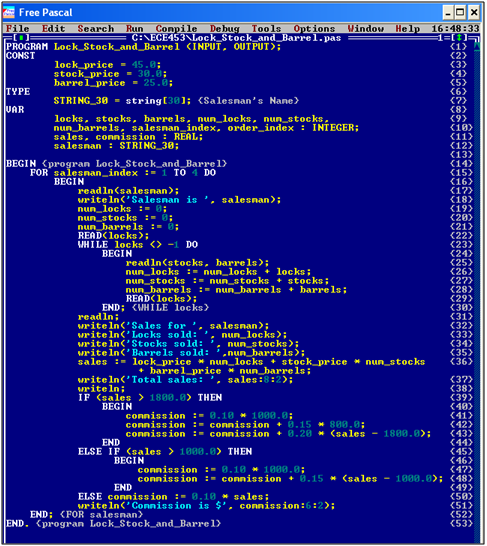• S(commission,48)
• {1-5,8-11,13,14,19-30,36,47,48,53}
• S(commission,40), S(commission,39), S(commission,38)
• {Ø}
• S(sales,35)
• {Ø}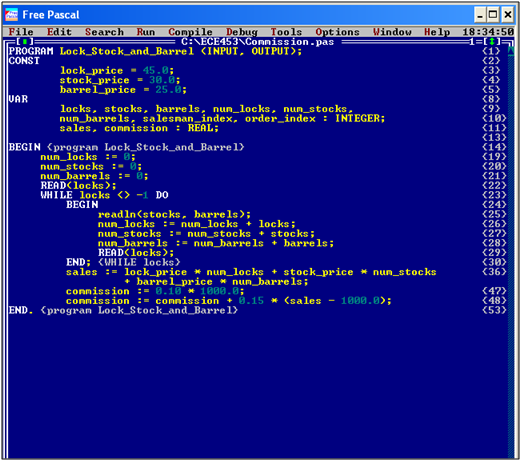• S(num_locks,34)
• {1,8,9,10,13,14,19, 22,23,24,26,29,30, 53}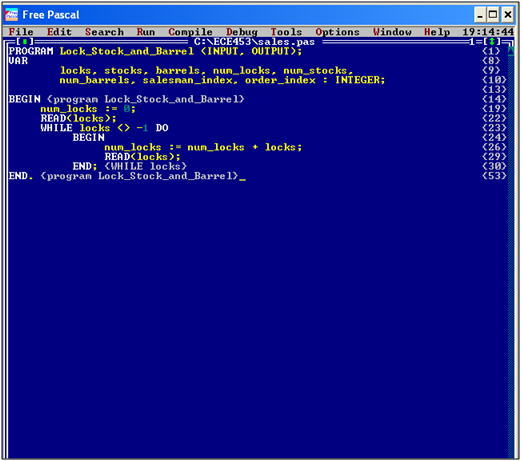• S(num_stocks,34)
• {1,8,9,10,13,14,20, 22-25,27,29,30,53}• S(num_barrels,34)
• {1,8,9,10,13,14,21-25,28,29,30,53}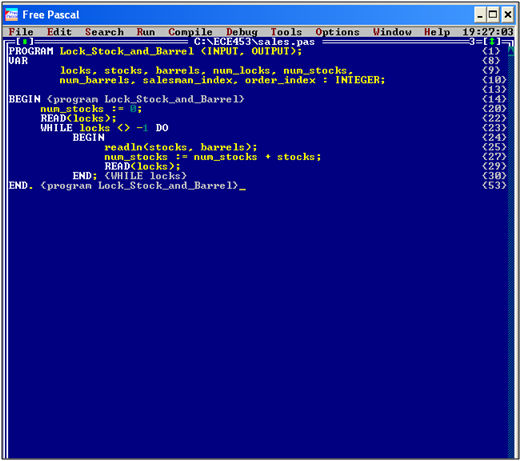• Find the program slice on FinalUse(low). Use the Program Dependency Graph approach
``````int binSearch(int x, int v[], int n)
{
int low = 0;
int high = n – 1;
int mid;
while (low <= high)
{
mid = (low + high) / 2;
if (x < v[mid])
high = mid – 1;
else if (x > v[mid])
low = mid + 1;
else
return mid;
}
return -1;
}                                                      ``````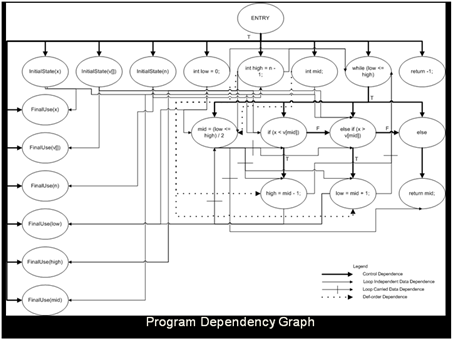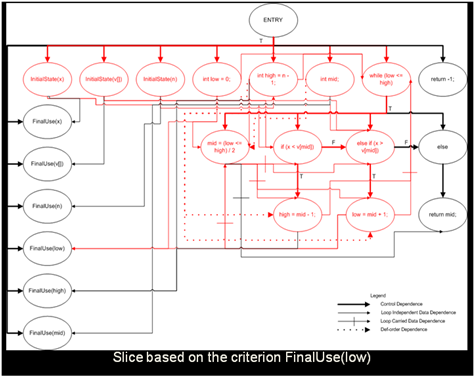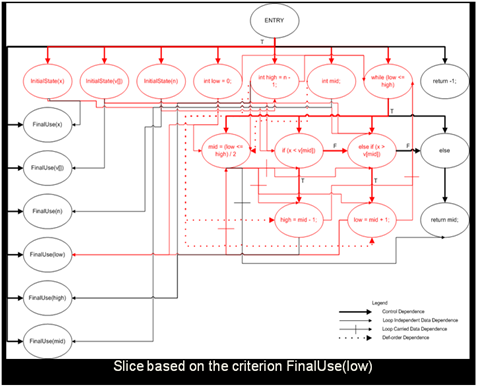``````int binSearch(int x, int v[], int n)
{
int low = 0;
int high = n – 1;
int mid;
while (low <= high)
{
mid = (low + high) / 2;
if (x < v[mid])
high = mid – 1;
else if (x > v[mid])
low = mid + 1;
else
return mid;
}
return -1;
}``````

Slice on FinalUse(low)

``````int binSearch(int x, int v[], int n)
{
int low = 0;
int high = n – 1;
int mid;
while (low <= high)
{
mid = (low + high) / 2;
if (x < v[mid])
high = mid – 1;
else if (x > v[mid])
low = mid + 1;
}
}``````